## Killing Vectors

If any set of points is displaced by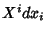where all distance relationships are unchanged (i.e., there is an Isometry), then the Vector field is called a Killing vector.(1)

so let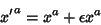(2)(3)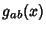(4)

where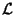is the Lie Derivative. An ordinary derivative can be replaced with a covariant derivative in a Lie Derivative, so we can take as the definition(5)(6)

which gives Killing's Equation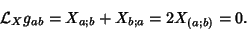(7)

A Killing vector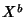satisfies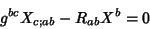(8)(9)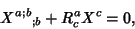(10)

where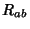is the Ricci Tensor andis the Riemann Tensor.

A 2-sphere with Metric(11)

has three Killing vectors, given by the angular momentum operators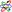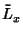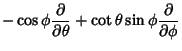(12)(13)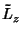(14)

The Killing vectors in Euclidean 3-space are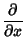(15)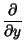(16)(17)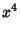(18)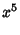(19)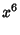(20)

In Minkowski Space, there are 10 Killing vectors(21)(22)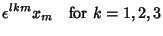(23)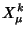(24)

The first group is Translation, the second Rotation, and the final corresponds to a boost.''